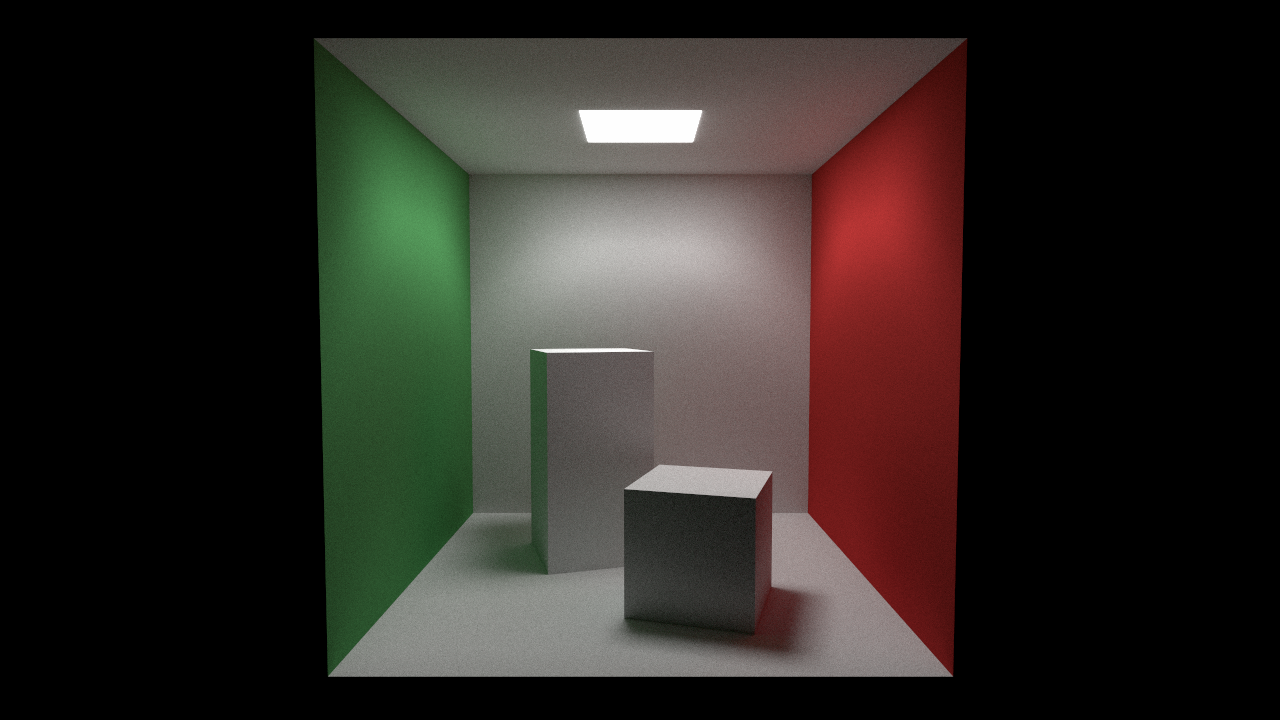In the last post, we implemented rectangles and rendered the Cornell Box scene. It was very satisfying but the program is way slow now. Let's look at some numbers:

• Previous scene with 8 spheres: 0.00009 ms/ray
• Cornell Box: 0.00132 ms/ray

We need to optimize rectangle intersection testing.

## Don't do anything more than you need to do

I hinted this in the last post. We have to get rid of matrix inverse function in the `IntersectWorld` function because it's too heavy. And guess what, we never need a non-inverted transform matrix in the intersection. So we can invert all rectangle's transform matrices in scene initialization and put an inverted matrix in the rectangle data. That is a pretty easy task to do. After this change, we get 0.000036 ms/ray. That is a huge boost up.

## SIMD Rectangles

We managed to test the intersection between a ray and multiple spheres with SIMD in the last posts. If you haven’t read the previous SIMD post, I encourage you to read it. Because this post gonna be the second part of that.

Okay, the idea is still the same: SIMD is not a computation problem. It simply is a memory layout problem. As long as our memory layouts are okay, the intersecton code will stay almost the same. Like the last time, we are thinking parallel. We have wide Vector3 struct that each element is a SIMD register and we used the AoSoA layout structure to map our sphere data. Rectangle struct has a Matrix4 type element. So, we need to make wide Vector4 and wide Matrix4 structs.

``````struct LaneVector4 {
LaneF32 x;
LaneF32 y;
LaneF32 z;
LaneF32 w;
};

struct LaneMatrix4 {
LaneVector4 data;
};
``````

These struct's variables and function implementations are the same as their scalar ones. Only they are using wide types now. Now, we have to load scene data into our wide types. Before implemented this, I thought this will generate nasty code. But turned out it wasn't.

``````for (uint32_t i = 0; i < rectangleLaneArrayCount; ++i) {
// Wide registers are like fixed-size arrays. If we think like that way,
// wide matrix becomes a 3D array and wide vector becomes a 2D array
// because every element in matrices and vectors are LANE_WIDTH size fixed array.
ALIGN_LANE float rectanglesTransformMatrix[LANE_WIDTH];
ALIGN_LANE float rectanglesNormal[LANE_WIDTH];
ALIGN_LANE float rectanglesMaterialIndex[LANE_WIDTH];

// Calculate the trail length
uint32_t remainingRectangles = rectangleCount - i * LANE_WIDTH;
uint32_t len = (remainingRectangles / LANE_WIDTH) > 0 ? LANE_WIDTH : remainingRectangles % LANE_WIDTH;
for (uint32_t j = 0; j < len; ++j) {
// Put scalar rectangle values into arrays
uint32_t rectangleIndex = j + i * LANE_WIDTH;
RectangleXY* rect = rectangles + rectangleIndex;

for (uint32_t rowIndex = 0; rowIndex < 4; ++rowIndex) {
for (uint32_t columnIndex = 0; columnIndex < 4; ++columnIndex) {
rectanglesTransformMatrix[rowIndex][columnIndex][j] = rect->transformMatrix[rowIndex][columnIndex];
}
}

rectanglesNormal[j] = rect->normal.x;
rectanglesNormal[j] = rect->normal.y;
rectanglesNormal[j] = rect->normal.z;
rectanglesMaterialIndex[j] = rect->materialIndex;
}

// Put those arrays into SIMD registers.
RectangleLane rectangleLane = {};
rectangleLane.transformMatrix = LaneMatrix4(rectanglesTransformMatrix);
rectangleLane.normal = LaneVector3(rectanglesNormal);
rectangleLane.materialIndex = LaneF32(rectanglesMaterialIndex);

// We are using the AoSoA layout. Our lane arrays aren't infinite like in the SoA layout.
// We need an array to put our wide rectangles
rectangleLaneArray[i] = rectangleLane;
}
``````

Now, we can convert the rectangle intersection code using wide variables:

``````for (uint32_t rectangleLaneIndex = 0; rectangleLaneIndex < world->rectangleLaneArrayCount; ++rectangleLaneIndex) {
RectangleLane* rectangleLane = world->rectangleLaneArray + rectangleLaneIndex;

// rectangle's transform matrix is inverted on scene initialization
LaneMatrix4 rayMatrix = rectangleLane->transformMatrix;
LaneVector3 localRayOrigin = (rayMatrix * LaneVector4(rayOriginLane, 1.0f)).xyz();
LaneVector3 localRayDirection = (rayMatrix * LaneVector4(rayDirectionLane, 0.0f)).xyz();

LaneF32 t = (-localRayOrigin.z) / localRayDirection.z;
LaneVector3 hitPoint = localRayOrigin + localRayDirection * t;

LaneF32 hit = hitPoint.x <= rectDefaultMaxPoint.x &
hitPoint.x >= rectDefaultMinPoint.x &
hitPoint.y <= rectDefaultMaxPoint.y &
hitPoint.y >= rectDefaultMinPoint.y;

LaneF32 hitMask = hit & (t < closestHitDistanceLane) & (t > minHitDistance);

LaneVector3 rectNormal = rectangleLane->normal;
// Check for incident ray direction vector direction
// If it's coming to back side of rectangle
// Flip the normal vector
LaneF32 dot = DotProduct(rectNormal, rayDirectionLane);
LaneVector3 flippedRectNormal = -rectNormal;
LaneF32 flipMask = dot > 0.0f;